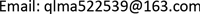1国家电网公司信息通信分公司，北京

2国网河北省电力公司信息通信分公司，河北 石家庄

3国网北京经济技术研究院，北京

4北京万可信息技术有限公司，北京1. 引言

2. 电力通信网诱因–故障告警关联分析

2.1. 电力通信业务承载交互模型分析

2.2. 业务驱动的故障关联分析

2.3. 电力通信网诱因与告警之间的关联性建模

∑ F k ∈ F | F k | = M (1)

P j G ( t ) = 1 − ∏ F k ∈ F G ( 1 − P k j F ( t ) ) (2)

P k j F ( t ) = ∑ i ∈ F k w i x i j P i ( t ) − ∑ i < l = 2 , i , l ∈ F k w i w l x i j x l j P i l ( t ) + ∑ i < l < r = 3 , i , l , r ∈ F k w i w l w r x i j x l j x r j P i l r ( t ) − ⋯ + ( − 1 ) h k − 1 ∏ i ∈ F k w i x i P 12 ⋯ h k ( t ) (3)

min Z 1 ( W ) = P k j F ( t ) (4)

∀ F k , ∑ i ∈ F k w i x i j ∂ P k j F ( t ) ∂ P i ( t ) = 1 (5)

min Z 2 ( W ) = − ∑ i ∈ F k w i x i j ∂ P k j F ( t ) ∂ P i ( t ) log 2 ( w i x i j ∂ P k j F ( t ) ∂ P i ( t ) ) (6)

min Z ( W ) = η Z 1 ( W ) + ( 1 − η ) Z 2 ( W ) s . t . { ∑ i ∈ F k w i x i j ∂ P k j F ( t ) ∂ P i ( t ) = 1 0 < w i < 1 (7)

2.3.1. 电力通信网故障与诱因关联优化模型简化

min Z 1 ( W ) = P k j F ( t ) ≐ ∑ i ∈ F k w i x i j P i ( t ) (8)

∂ P k j F ( t ) ∂ P i ( t ) ≐ ∂ ∑ i ∈ F k w i x i j P i ( t ) ∂ P i ( t ) = w i (9)

min Z ( W ) = − η ∑ i ∈ F k x i j w i 2 log 2 ( x i j w i 2 ) + ( 1 − η ) ( ∑ i ∈ F k w i x i j P i ( t ) ) s . t . { ∑ i ∈ F k x i j w i 2 = 1 0 < w i < 1 (10)

2.3.2. 扩展的牛顿迭代法

2.3.3. 实例分析

² 自然因素(F1)：地震，强风，老鼠啃咬，火灾；

² 人为因素(F2)：挪动光纤，施工挖断；

² 设备因素(F3)：自然老化。

1) 首先，对自然因素的诱因概率进行建模，设地震级别为1~10级，强风分为1~12级，老鼠啃咬程度为1~4级，火灾分为1~5级。设地震的起始影响等级为4级，强风为6级，老鼠啃咬为2级，火灾为1级，此时正遭遇8级台风和1级老鼠啃咬危险。

2) 对于人为因素，设挪动光纤的级别只有1，施工挖断的级别为2，两种因素的到达率分别为0.001和0.002，当前挪动光纤存在，且施工存在1级挖断影响可能性，因此则可以得到施工因素的发生概率分别为1−e −0.002和0.5 (1−e−0.01)。

3) 对于设备因素，设其使用时间为3年，使用寿命为5年，对应的发生故障概率为1−0.4e−3

min Ζ ( W ) = − ( w 2 2 log 2 w 2 2 + w 3 2 log 2 w 3 2 ) + 0.5 ( 0. 33 w 2 e − 2 + 0. 33 w 3 e − 4 )

3. 业务风险模型构建

3.1. 业务状态分析

3.2. 故障告警影响级别模型

E i = P i ⋅ D i (11)

D i = w i × v i h × C i (12)

vih为业务i所属种类h的权重。wi为业务si承载的设备和链路的权重和。Ci为业务权重，可参考文献  。

(13)

4. 基于仿真的告警–故障关联风险分析实例

5. 结语

2017年国家电网公司总部科技项目：系统保护通信网络技术体制及关键技术研究(Grant No. SGXT0000ASJS1700054)。